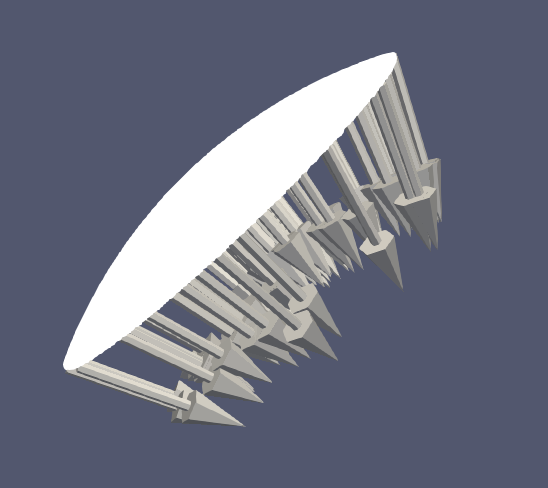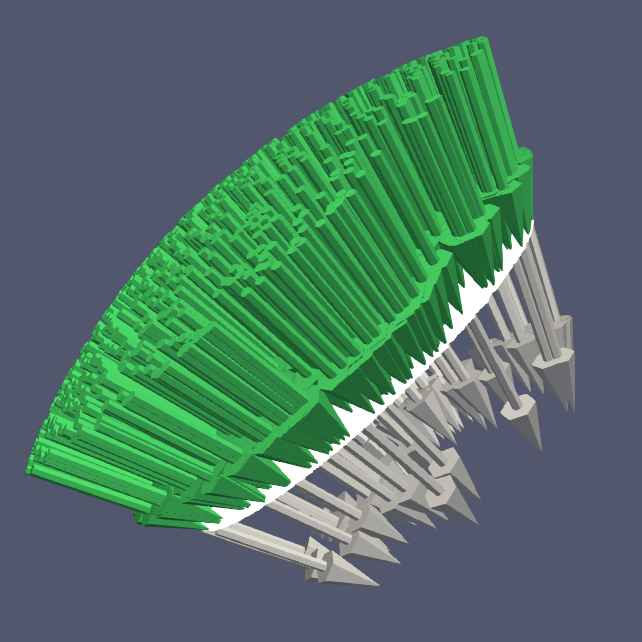I want to visualize vector fields in paraview. However, paraview has the property that it shows the vectors with the tail attached to the vertex at which they are specified. While this might be okay if you plot say velocity fields, it might be weird to look at when plotting force vectors acting on some surface vertices.

In this case, the vectors show inward into the surface, which is a bit confusing:Thus, it would be nice to have the vectors pointing their tip at the specified node. Unfortunately, paraview does not seem to have a setting to do this automatically.

However, there is a trick to make it work! First of all, you have to invert the direction of the vector by using the calculator. Add a new Calculator filter, use Attribute Type "Point Data", select the vector and use the equation `-dir` (In my case the vector is called `dir`).

Then apply a new Glyph filter, however under the advanced properties, select "Invert":Please find a paraview state file as an example here: